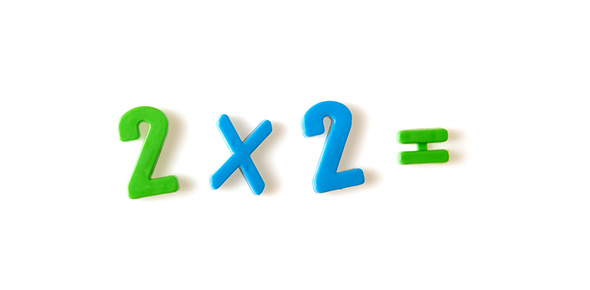# Principles Of Multiplication

10 Questions | Total Attempts: 245SettingsIn this quiz, we’ll be looking at the principles of multiplication – a counting principle which theorises that if there are A ways of doing something and B ways of doing another, there must be AB ways of doing both.

• 1.
Changing the order of two numbers in a multiplication equation. (e.g., 7 x 9 = 9 x 7).
• A.

Distributive Law

• B.

Multiplication Law

• C.

Commutative Law

• 2.
A x (b + c) = a x b + a x c or vice versa. (e.g., “9 times 14” is 9 x (7 + 7) = 9 x 7 + 9 x 7).
• A.

Distributive Law

• B.

Multiplication Law

• C.

Commutative Law

• 3.
Basic multiplication facts are considered to be ______________or furtheradvancement in mathematics.
• 4.
8 x 9
• A.

17

• B.

56

• C.

72

• 5.
6 x 6
• A.

12

• B.

36

• C.

24

• 6.
9 x 5
• A.

45

• B.

40

• C.

50

• 7.
10 x 10 = 110
• A.

True

• B.

False

• 8.
5 x 5 = 25
• A.

True

• B.

False

• 9.
Students frequently find_______________tasks to be a stumbling block in their mathematical progress.
• 10.
2 x 4 = 8
• A.

True

• B.

False

Related Topics